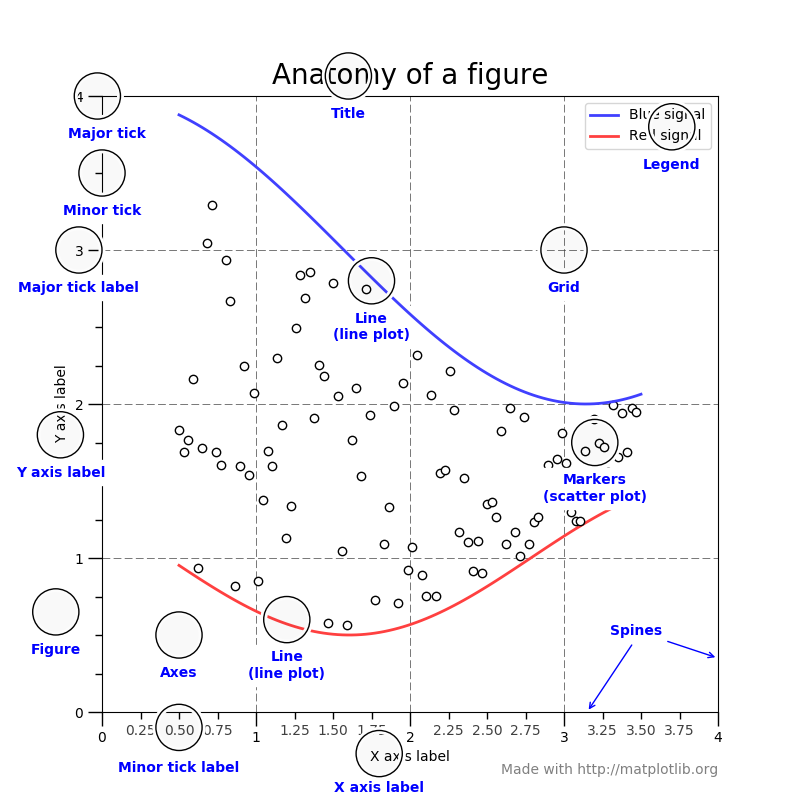# Getting Stared with Matplotlib - Adding Title and Axis Label to Charts using Pyplot

Now we will learn how to add various elements to our plots.

Every plot in Matplotlib consists of various elements. If is shown extensively in the image below:Note: The image, Anatomy of Figure, was obtained from the official Matplotlib website.

INSTRUCTIONS
• We will start by adding a title and axis labels to our plot. To add the title, we use the `title` method:

``````import numpy as np

X = np.array([1, 2, 5, 8, 12])
Y = np.array([3, 6, 7, 12, 9])

import matplotlib.pyplot as plt
plt.plot(X, Y)
plt.<< your code goes here >>("This is the title")
``````
• And, for the the axis labels, we use the `xlabel` and `ylabel` methods respectively:

``````plt.xlabel("X label")
plt.<< your code goes here >>("Y label")
``````
• Finally, we use `show` to display our plot:

``````plt.<< your code goes here >>
``````

No hints are availble for this assesment

Answer is not availble for this assesment

Note - Having trouble with the assessment engine? Follow the steps listed here